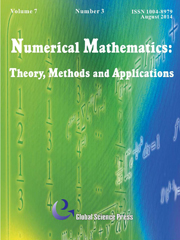Home
Hostname: page-component-846f6c7c4f-tkmnj Total loading time: 0.271 Render date: 2022-07-06T21:45:15.063Z Has data issue: true Feature Flags: { "shouldUseShareProductTool": true, "shouldUseHypothesis": true, "isUnsiloEnabled": true, "useRatesEcommerce": false, "useNewApi": true } hasContentIssue trueNumerical Mathematics: Theory, Methods and Applications

# Spectral Optimization Methods for the Time Fractional Diffusion Inverse Problem

Published online by Cambridge University Press:  28 May 2015

*

## Abstract

An inverse problem of reconstructing the initial condition for a time fractional diffusion equation is investigated. On the basis of the optimal control framework, the uniqueness and first order necessary optimality condition of the minimizer for the objective functional are established, and a time-space spectral method is proposed to numerically solve the resulting minimization problem. The contribution of the paper is threefold: 1) a priori error estimate for the spectral approximation is derived; 2) a conjugate gradient optimization algorithm is designed to efficiently solve the inverse problem; 3) some numerical experiments are carried out to show that the proposed method is capable to find out the optimal initial condition, and that the convergence rate of the method is exponential if the optimal initial condition is smooth.

Type
Research Article
Information

## Access options

Get access to the full version of this content by using one of the access options below. (Log in options will check for institutional or personal access. Content may require purchase if you do not have access.)

## References

 Canuto, C., Hussaini, M. Y., Quarteroni, A. and Zang, T. A., Spectral Methods Fundamentals in Single Domains, Springer-Verlag, Berlin, 2006.Google Scholar
 Cheng, J., Nakagawa, J., Yamamoto, M. and Yamazaki, T., Uniqueness in an inverse problem for a one-dimensional fractional diffusion equation, Inverse Problems, 25(11) (2009), pp. 115002.CrossRefGoogle Scholar
 V Ervin, J. and Roop, J. P., Variational formulation for the stationary fractional advection dispersion equation, Numer. Methods Partial Differential Equations, 22(3) (2006), pp. 558576.CrossRefGoogle Scholar
 V Ervin, J. and Roop, J. P., Variational solution of fractional advection dispersion equations on bounded domains in R d , Numer. Methods Partial Differential Equations, 23(2) (2007), pp. 256281.CrossRefGoogle Scholar
 Isakov, V., Inverse Problems for Partial Differential Equations, Springer Science, New York, 2006.Google Scholar
 Langlands, T. A.M. and Henry, B. I., The accuracy and stability of an implicit solution method for the fractional diffusion equation, J. Comput. Phys., 205(2) (2005), pp. 719736.CrossRefGoogle Scholar
 Li, Xianjuan and Xu, Chuanju, A space-time spectral method for the time fractional diffusion equation, SIAM J. Numer. Anal., 47(3) (2009), pp. 21082131.CrossRefGoogle Scholar
 Lin, Yumin and Xu, Chuanju, Finite difference/spectral approximations for the time-fractional diffusion equation, J. Comput. Phys., 225(2) (2007), pp. 15331552.CrossRefGoogle Scholar
 Lions, J. L., Optimal Control of Systems Governed by Partial Differential Equations, volume 170 of Grundlehren Math. Wiss., Springer-Verlag, Berlin, 1971.Google Scholar
 Liu, F., Shen, S., Anh, V and Turner, I., Analysis of a discrete non-markovian random walk approximation for the time fractional diffusion equation, ANZIAM J, 46(4) (2005), pp. 488504.CrossRefGoogle Scholar
 Luchko, Y., Maximum principle for the generalized time-fractional diffusion equation, J. Math. Anal. Appl, 351(1) (2009), pp. 218223.CrossRefGoogle Scholar
 Luchko, Y., Some uniqueness and existence results for the initial-boundary-value problems for the generalized time-fractional diffusion equation, Comput. Math. Appl, 59(5) (2010), pp. 17661772.CrossRefGoogle Scholar
 Podlubny, I., Fractional Differential Equations, Acad. Press, New York, 1999.Google Scholar
 Roop, J. P., Computational aspects of FEM approximation of fractional advection dispersion equations on bounded domains in Ш2 , J. Comput. Appl. Math., 193(1) (2006), pp. 243268.CrossRefGoogle Scholar
 Sakamoto, K. and Yamamoto, M., Initial value/boundary value problems for fractional diffusion-wave equations and applications to some inverse problems, J. Math. Anal. Appl., 382(1) (2011), pp. 426447.CrossRefGoogle Scholar
 Samarskii, A. A. and Vabishchevich, P. N., Numerical Methods for Solving Inverse Problems of Mathematical Physics, De Gruyter, Berlin, 2007.CrossRefGoogle Scholar
 Schneider, W.R. and Wyss, W., Fractional diffusion and wave equations, J. Math. Phys, 30(1) (1989), pp. 134144.CrossRefGoogle Scholar
 Sun, Z. and Wu, X., A fully discrete difference scheme for a diffusion-wave system, Appl. Numer. Math., 56(2) (2006), pp. 193209.CrossRefGoogle Scholar
 Wyss, W., The fractional diffusion equation, J. Math. Phys., 27(11) (1986), pp. 27822785.CrossRefGoogle Scholar
 Zhang, Y. and Xu, X., Inverse source problem for a fractional diffusion equation, Inverse Problems, 27(3) (2011), pp. 035010.CrossRefGoogle Scholar

# Save article to Kindle

Note you can select to save to either the @free.kindle.com or @kindle.com variations. ‘@free.kindle.com’ emails are free but can only be saved to your device when it is connected to wi-fi. ‘@kindle.com’ emails can be delivered even when you are not connected to wi-fi, but note that service fees apply.

Find out more about the Kindle Personal Document Service.

Spectral Optimization Methods for the Time Fractional Diffusion Inverse Problem
Available formats
×

# Save article to Dropbox

To save this article to your Dropbox account, please select one or more formats and confirm that you agree to abide by our usage policies. If this is the first time you used this feature, you will be asked to authorise Cambridge Core to connect with your Dropbox account. Find out more about saving content to Dropbox.

Spectral Optimization Methods for the Time Fractional Diffusion Inverse Problem
Available formats
×

# Save article to Google Drive

To save this article to your Google Drive account, please select one or more formats and confirm that you agree to abide by our usage policies. If this is the first time you used this feature, you will be asked to authorise Cambridge Core to connect with your Google Drive account. Find out more about saving content to Google Drive.

Spectral Optimization Methods for the Time Fractional Diffusion Inverse Problem
Available formats
×
×